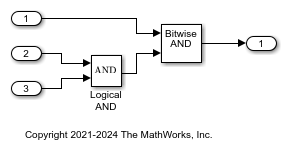# Control Operator Type in Generated Code

This example shows how to optimize the generated code by controlling the operator type in the code. You can generate code with Bitwise or Logical operators or a combination of both for inputs with Boolean data type. Selecting bitwise operators in the code might improve ROM efficiency when the code contains bitwise operators.

When you select a value for the Operator to represent Bitwise and Logical Operator blocks parameter, the generated code maintains the operators as modelled or converts all operators into either bitwise or logical operators independent of the modeling blocks.

NOTE: This example requires Embedded Coder®.

### Example Model

In the model matlab:logicalandbitwise, a Logical AND block connects to a Bitwise AND block.

```model = 'logicalandbitwise'; open_system(model); ```### Generate Code as Modeled

Use your system temporary folder for building and inspection process.

```currentDir = pwd; ```
1. Open the Configuration Parameters dialog box.

2. On the Optimization pane, set the Operator to represent Bitwise and Logical Operator blocks parameter to `Same as modeled`.

Alternatively, use the command-line API to set the parameter value:

```set_param(model, 'BitwiseOrLogicalOp', 'Same as modeled'); evalc('slbuild(model)'); ```

View the generated code with operators in the code that matches the modeled blocks. Here is a portion of `logicalandbitwise.c`.

```cfile = fullfile(currentDir,'logicalandbitwise_ert_rtw','logicalandbitwise.c'); rtwdemodbtype(cfile,'/* Model step function ','/* Model initialize function',1, 1); ```
```/* Model step function */ void logicalandbitwise_step(void) { /* Outport: '<Root>/Out1' incorporates: * Inport: '<Root>/Input' * Inport: '<Root>/Input1' * Inport: '<Root>/Input2' * Logic: '<Root>/Logical AND' * S-Function (sfix_bitop): '<Root>/Bitwise AND' */ logicalandbitwise_Y.Out1 = (logicalandbitwise_U.Input1 && logicalandbitwise_U.Input2) & logicalandbitwise_U.Input; } ```

The generated code contains both a logical `&&` operator and a bitwise `&` operator representing the Logical AND block and Bitwise AND block in the code.

### Generate Code with Logical Operators

1. Open the Configuration Parameters dialog box.

2. On the Optimization pane, set the Operator to represent Bitwise and Logical Operator blocks parameter to `Logical operator`.

Alternatively, use the command-line API to set the parameter value:

```set_param(model, 'BitwiseOrLogicalOp', 'Logical operator'); evalc('slbuild(model)'); ```

View the generated code with only logical operators. Here is a portion of `logicalandbitwise.c`.

```cfile = fullfile(currentDir,'logicalandbitwise_ert_rtw','logicalandbitwise.c'); rtwdemodbtype(cfile,'/* Model step function ','/* Model initialize function',1, 1); ```
```/* Model step function */ void logicalandbitwise_step(void) { /* Outport: '<Root>/Out1' incorporates: * Inport: '<Root>/Input' * Inport: '<Root>/Input1' * Inport: '<Root>/Input2' * Logic: '<Root>/Logical AND' * S-Function (sfix_bitop): '<Root>/Bitwise AND' */ logicalandbitwise_Y.Out1 = (logicalandbitwise_U.Input && (logicalandbitwise_U.Input1 && logicalandbitwise_U.Input2)); } ```

The generated code contains only the logical `&&` operator. The Bitwise AND block in the model is converted into the logical `&&` operator in the code.

### Generate Code with Bitwise Operators

1. Open the Configuration Parameters dialog box.

2. On the Optimization pane, set the Operator to represent Bitwise and Logical Operator blocks parameter to `Bitwise operator`.

Alternatively, use the command-line API to set the parameter value:

```set_param(model, 'BitwiseOrLogicalOp', 'Bitwise operator'); evalc('slbuild(model)'); ```

View the generated code with only bitwise operators. Here is a portion of `logicalandbitwise.c`.

```cfile = fullfile(currentDir,'logicalandbitwise_ert_rtw','logicalandbitwise.c'); rtwdemodbtype(cfile,'/* Model step function ','/* Model initialize function',1, 1); ```
```/* Model step function */ void logicalandbitwise_step(void) { /* Outport: '<Root>/Out1' incorporates: * Inport: '<Root>/Input' * Inport: '<Root>/Input1' * Inport: '<Root>/Input2' * Logic: '<Root>/Logical AND' * S-Function (sfix_bitop): '<Root>/Bitwise AND' */ logicalandbitwise_Y.Out1 = (boolean_T)(logicalandbitwise_U.Input1 & logicalandbitwise_U.Input2) & logicalandbitwise_U.Input; } ```

The generated code contains only the bitwise `&` operator. The Logical AND block in the model is converted into the bitwise `&` operator in the code.

Close the model and code generation report.

```bdclose(model) rtwdemoclean; cd(currentDir) ```